## Marginal and conditional densities

Although the posterior density given by (1) provides all that is needed for inference about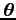, there may be particular interest in a subset of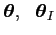of dimension2. where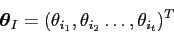(6)

and, ifis of dimension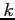,(7)

Denoting the complement of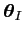with respect toas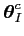, then the Bayesian paradigm gives inference foras the marginal posterior density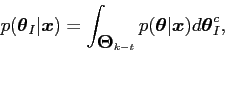(8)

where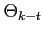is the parameter space supporting, i.e. the appropriate region of integration for the subsetof.

In a similar way, inference about, whenare known, is given by the conditional posterior density(9)

danny 2009-07-23